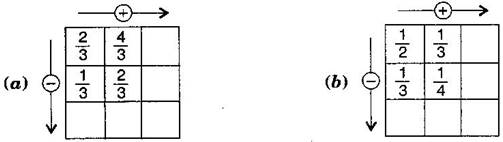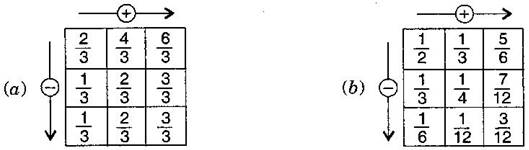1. /
2. CBSE
3. /
4. Class 06
5. /
6. Mathematics
7. /
8. NCERT Solutions for Class...

# NCERT Solutions for Class 6 Maths Exercise 7.6

NCERT solutions for Maths Fractions

## NCERT Solutions for Class 6 Maths Fractions

###### Question 1.Solve:

(a) {tex}\frac{2}{3} + \frac{1}{7}{/tex}

(b) {tex}\frac{3}{{10}} + \frac{7}{{15}}{/tex}

(c) {tex}\frac{4}{9} + \frac{2}{7}{/tex}

(d) {tex}\frac{5}{7} + \frac{1}{3}{/tex}

(e) {tex}\frac{2}{5} + \frac{1}{6}{/tex}

(f) {tex}\frac{4}{5} + \frac{2}{3}{/tex}

(g) {tex}\frac{3}{4} – \frac{1}{3}{/tex}

(h) {tex}\frac{5}{6} – \frac{1}{3}{/tex}

(i) {tex}\frac{2}{3} + \frac{3}{4} + \frac{1}{2}{/tex}

(j) {tex}\frac{1}{2} + \frac{1}{3} + \frac{1}{6}{/tex}

(k) {tex}1\frac{1}{3} + 3\frac{2}{3}{/tex}

(l) {tex}4\frac{2}{3} + 3\frac{1}{4}{/tex}

(m) {tex}\frac{{16}}{5} – \frac{7}{5}{/tex}

(n) {tex}\frac{4}{3} – \frac{1}{2}{/tex}

(a) L.C.M. of 3 and 7 is 21

{tex}\therefore {/tex} {tex}\frac{2}{3} + \frac{1}{7} = \frac{{2 \times 7 + 1 \times 3}}{{21}} = \frac{{14 + 3}}{{21}} = \frac{{17}}{{21}}{/tex}

(b) L.C.M. of 10 and 15 is 30

{tex}\therefore {/tex} {tex}\frac{3}{{10}} + \frac{7}{{15}} = \frac{{3 \times 3 + 7 \times 2}}{{30}} = \frac{{9 + 14}}{{30}} = \frac{{23}}{{30}}{/tex}

(c) L.C.M. of 9 and 7 is 63

{tex}\therefore {/tex} {tex}\frac{4}{9} + \frac{2}{7} = \frac{{4 \times 7 + 2 \times 9}}{{63}} = \frac{{28 + 18}}{{63}} = \frac{{46}}{{63}}{/tex}

(d)L.C.M. of 7 and 3 is 21

{tex}\therefore {/tex} {tex}\frac{5}{7} + \frac{1}{3} = \frac{{5 \times 3 + 7 \times 1}}{{21}} = \frac{{15 + 7}}{{21}} = \frac{{22}}{{21}} = 1\frac{1}{{21}}{/tex}

(e)L.C.M. of 5 and 6 is 30

{tex}\therefore {/tex} {tex}\frac{2}{5} + \frac{1}{6} = \frac{{2 \times 6 + 5 \times 1}}{{30}} = \frac{{12 + 5}}{{30}} = \frac{{17}}{{30}}{/tex}

(f)L.C.M. of 5 and 3 is 15

{tex}\therefore {/tex} {tex}\frac{4}{5} + \frac{2}{3}{/tex} = {tex}\frac{{4 \times 3 + 2 \times 5}}{{15}} = \frac{{12 + 10}}{{15}} = \frac{{22}}{{15}} = 1\frac{7}{{15}}{/tex}

(g)L.C.M. of 4 and 3 is 12

{tex}\therefore {/tex} {tex}\frac{3}{4} – \frac{1}{3} = \frac{{3 \times 3 – 4 \times 1}}{{12}} = \frac{{9 – 4}}{{12}} = \frac{5}{{12}}{/tex}

(h)L.C.M. of 6 and 3 is 6

{tex}\therefore {/tex}

{tex}\frac{5}{6}-\frac{1}{3}=\frac{5\times 1-2\times 1}{6}=\frac{5-2}{6}=\frac{3}{6}=\frac{1}{2}{/tex}

(i)L.C.M. of 3, 4 and 2 is 12

{tex}\therefore {/tex} {tex}\frac{2}{3} + \frac{3}{4} + \frac{1}{2} = \frac{{2 \times 4 + 3 \times 3 + 1 \times 6}}{{12}} = \frac{{6 + 9 + 6}}{{12}} = \frac{{23}}{{12}} = 1\frac{{11}}{{12}}{/tex}

(j)L.C.M. of 2, 3, and 6 is 6

{tex}\therefore {/tex}

{tex}\frac{1}{2}+\frac{1}{3}+\frac{1}{6}=\frac{1\times 3+1\times 2+1\times 1}{6}=\frac{3+2+1}{6}=\frac{6}{6}=1{/tex}

(k)L.C.M. of 3 and 3 is 3

{tex}\therefore {/tex}

{tex}\frac{4}{3}+\frac{11}{3}=\frac{4+11}{3}=\frac{15}{3}=5{/tex}

(l)L.C.M. of 3 and 4 is 12

{tex}\therefore {/tex} {tex}\frac{{14}}{3} + \frac{{13}}{4} = \frac{{14 \times 4 + 13 \times 3}}{{12}} = \frac{{56 + 39}}{{12}} = \frac{{95}}{{12}} = 7\frac{{11}}{{12}}{/tex}

(m)L.C.M. of 5 and 5 is 5

{tex}\therefore {/tex} {tex}\frac{{16}}{5} – \frac{7}{5} = \frac{{16 – 7}}{5} = \frac{9}{5} = 1\frac{4}{5}{/tex}

(n)L.C.M. of 3 and 2 is 6

{tex}\therefore {/tex} {tex}\frac{4}{3} – \frac{1}{2} = \frac{{4 \times 2 – 1 \times 3}}{6} = \frac{{8 – 3}}{6} = \frac{5}{6}{/tex}

NCERT Solutions for Class 6 Maths Exercise 7.6

###### Question 2.Sarika bought {tex}\frac{2}{5}{/tex} meter of ribbon and Lalita {tex}\frac{3}{4}{/tex} meter of ribbon. What is the total length of the ribbon they bought?

Ribbon bought by Sarita = {tex}\frac{2}{5}{/tex} m and Ribbon bought by Lalita = {tex}\frac{3}{4}{/tex} m

Total length of ribbon = {tex}\frac{2}{5} + \frac{3}{4}{/tex} = {tex}\frac{{2 \times 4 + 5 \times 3}}{{20}}{/tex} [{tex}\because {/tex} L.C.M. of 5 and 4 is 20]

= {tex}\frac{{8 + 15}}{{20}} = \frac{{23}}{{20}} = 1\frac{3}{{20}}{/tex} m

Therefore, they bought {tex}1\frac{3}{{20}}{/tex} m of ribbon.

NCERT Solutions for Class 6 Maths Exercise 7.6

###### Question 3.Naina was given {tex}1\frac{1}{2}{/tex} piece of cake and Najma was given {tex}1\frac{1}{3}{/tex} piece of cake. Find the total amount of cake given to both of them.

Cake taken by Naina = {tex}1\frac{1}{2}{/tex} piece and Cake taken by Najma = {tex}1\frac{1}{3}{/tex} piece

Total cake taken = {tex}1\frac{1}{2}{/tex} + {tex}1\frac{1}{3}{/tex} = {tex}\frac{3}{2} + \frac{4}{3}{/tex} = {tex}\frac{{3 \times 3 + 4 \times 2}}{6}{/tex} [{tex}\because {/tex} L.C.M. of 2 and 3 is 6]

= {tex}\frac{{9 + 8}}{6} = \frac{{17}}{6} = 2\frac{5}{6}{/tex}

Therefore total consumption of cake is {tex}2\frac{5}{6}{/tex}.

###### Question 4.Fill in the boxes:

(a) {tex}\boxed{{\text{ }}} – \frac{5}{8} = \frac{1}{4}{/tex}

(b) {tex}\boxed{{\text{ }}} – \frac{1}{5} = \frac{1}{2}{/tex}

(c) {tex}\frac{1}{2} – \boxed{{\text{ }}} = \frac{1}{6}{/tex}

(a) {tex}\frac{1}{4} + \frac{5}{8} = \frac{{2 + 5}}{8} = \frac{7}{8}{/tex}

(b) {tex}\frac{1}{2} + \frac{1}{5} = \frac{{5 + 2}}{{10}} = \frac{7}{{10}}{/tex}

(c) {tex}\frac{1}{2} – \frac{1}{6} = \frac{{3 – 1}}{6} = \frac{2}{6}{/tex}

NCERT Solutions for Class 6 Maths Exercise 7.6

###### Question 5.Complete the addition – subtraction box:Sol.###### Question 6.A piece of wire {tex}\frac{7}{8}{/tex} meter long broke into two pieces. One piece was {tex}\frac{1}{4}{/tex} meter long. How long is the other piece?

Total length of wire = {tex}\frac{7}{8}{/tex} meter

Length of first part = {tex}\frac{1}{4}{/tex} meter

Remaining part = {tex}\frac{7}{8} – \frac{1}{4} = \frac{{7 \times 1 – 2 \times 1}}{8}{/tex} [{tex}\because {/tex} L.C.M. of 8 and 4 is 8]

= {tex}\frac{{7 – 2}}{8} = \frac{5}{8}{/tex} meter

Therefore, the length of remaining part is {tex}\frac{5}{8}{/tex} meter.

NCERT Solutions for Class 6 Maths Exercise 7.6

###### Question 7.Nandini house is {tex}\frac{9}{{10}}{/tex} km from her school. She walked some distance and then took a bus for {tex}\frac{1}{2}{/tex} km to reach the school. How far did she walk?

Total distance between school and house = {tex}\frac{9}{{10}}{/tex} km

Distance covered by bus = {tex}\frac{1}{2}{/tex} km

Remaining distance = {tex}\frac{9}{{10}} – \frac{1}{2} = \frac{{9 \times 1 – 1 \times 5}}{{10}}{/tex} [{tex}\because {/tex} L.C.M. of 10 and 2 is 10]

= {tex}\frac{9-5}{10}=\frac{4}{10}=\frac{2}{5}{/tex} km

Therefore, distance covered by walking us {tex}\frac{2}{5}{/tex} km.

###### Question 8.Ahsa and Samuel have bookshelves of the same size partly filled with books. Asha’s shelf is {tex}\frac{5}{6}th{/tex} full and Samuel’s shelf is {tex}\frac{2}{5}th{/tex} full. Whose bookshelf is more full? By what fraction?

{tex}\frac{5}{6}{/tex} and {tex}\frac{2}{5}{/tex}

{tex} \Rightarrow {/tex} {tex}\frac{5}{6} \times \frac{5}{5} = \frac{{25}}{{30}}{/tex} and {tex}\frac{2}{5} \times \frac{6}{6} = \frac{{12}}{{30}}{/tex} [{tex}\because {/tex} L.C.M. of 6 and 5 is 30]

{tex}\because {/tex} {tex}\frac{{25}}{{30}} > \frac{{12}}{{30}}{/tex} {tex} \Rightarrow {/tex} {tex}\frac{5}{6} > \frac{2}{5}{/tex}

{tex}\therefore {/tex} Asha’s bookshelf is more covered than Samueal.

Difference = {tex}\frac{{25}}{{30}} – \frac{{12}}{{30}} = \frac{{13}}{{30}}{/tex}

NCERT Solutions for Class 6 Maths Exercise 7.6

###### Question 9.Jaidev takes {tex}2\frac{1}{5}{/tex} minutes to walk across the school ground. Rahul takes {tex}\frac{7}{4}{/tex} minutes to do same. Who takes less time and by what fraction?

Time taken by jaidev = {tex}2\frac{1}{5}{/tex} minutes = {tex}\frac{{11}}{5}{/tex} minutes

Time taken by Rahul = {tex}\frac{7}{4}{/tex} minutes

Difference = {tex}\frac{{11}}{5} – \frac{7}{4}{/tex} = {tex}\frac{{11 \times 4 – 7 \times 5}}{{20}}{/tex} [{tex}\because {/tex} L.C.M. of 5 and 4 is 20]

= {tex}\frac{{44 – 35}}{{20}} = \frac{9}{{20}}{/tex} minutes

Thus, Rahul takes less time, which is {tex}\frac{9}{{20}}{/tex} minutes.

## NCERT Solutions for Class 6 Maths Exercise 7.6

NCERT Solutions Class 6 Maths PDF (Download) Free from myCBSEguide app and myCBSEguide website. Ncert solution class 6 Maths includes text book solutions from Class 6 Maths Book . NCERT Solutions for CBSE Class 6 Maths have total 14 chapters. 6 Maths NCERT Solutions in PDF for free Download on our website. Ncert Maths class 6 solutions PDF and Maths ncert class 6 PDF solutions with latest modifications and as per the latest CBSE syllabus are only available in myCBSEguide.

## CBSE app for Students

To download NCERT Solutions for Class 6 Maths, Social Science Computer Science, Home Science, Hindi English, Maths Science do check myCBSEguide app or website. myCBSEguide provides sample papers with solution, test papers for chapter-wise practice, NCERT solutions, NCERT Exemplar solutions, quick revision notes for ready reference, CBSE guess papers and CBSE important question papers. Sample Paper all are made available through the best app for CBSE students and myCBSEguide website.Test Generator

Create question paper PDF and online tests with your own name & logo in minutes.myCBSEguide

Question Bank, Mock Tests, Exam Papers, NCERT Solutions, Sample Papers, Notes